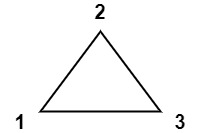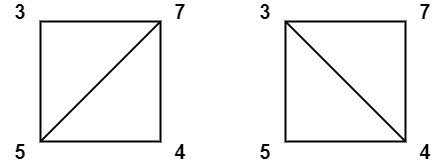## Algorithm

Problem Name: 1039. Minimum Score Triangulation of Polygon

You have a convex `n`-sided polygon where each vertex has an integer value. You are given an integer array `values` where `values[i]` is the value of the `ith` vertex (i.e., clockwise order).

You will triangulate the polygon into `n - 2` triangles. For each triangle, the value of that triangle is the product of the values of its vertices, and the total score of the triangulation is the sum of these values over all `n - 2` triangles in the triangulation.

Return the smallest possible total score that you can achieve with some triangulation of the polygon.

Example 1:```Input: values = [1,2,3]
Output: 6
Explanation: The polygon is already triangulated, and the score of the only triangle is 6.
```

Example 2:```Input: values = [3,7,4,5]
Output: 144
Explanation: There are two triangulations, with possible scores: 3*7*5 + 4*5*7 = 245, or 3*4*5 + 3*4*7 = 144.
The minimum score is 144.
```

Example 3:```Input: values = [1,3,1,4,1,5]
Output: 13
Explanation: The minimum score triangulation has score 1*1*3 + 1*1*4 + 1*1*5 + 1*1*1 = 13.
```

Constraints:

• `n == values.length`
• `3 <= n <= 50`
• `1 <= values[i] <= 100`

## Code Examples

### #1 Code Example with Java Programming

```Code - Java Programming```

``````
class Solution {
public int minScoreTriangulation(int[] A) {
int n = A.length;
int[][] dp = new int[n][n];
for (int d = 2; d < n; ++d) {
for (int i = 0; i + d < n; ++i) {
int j = i + d;
dp[i][j] = Integer.MAX_VALUE;
for (int k = i + 1; k < j; ++k) {
dp[i][j] = Math.min(dp[i][j], dp[i][k] + dp[k][j] + A[i] * A[j] * A[k]);
}
}
}
return dp[n - 1];
}
}
``````
Copy The Code &

Input

cmd
values = [1,2,3]

Output

cmd
6

### #2 Code Example with Javascript Programming

```Code - Javascript Programming```

``````
const minScoreTriangulation = function(A) {
if(A.length <= 2) return 0
if(A.length === 3) return A * A * A
return chk(A, A.length)
};

function cost(points, i, j, k) {
let p1 = points[i],
p2 = points[j],
p3 = points[k]
return p1 * p2 * p3
}

function chk(points, n) {
if (n < 3) return 0

const table = Array.from({ length: n }, () => new Array(n).fill(0))

for (let gap = 0; gap < n; gap++) {
for (let i = 0, j = gap; j < n; i++, j++) {
if (j < i + 2) table[i][j] = 0
else {
table[i][j] = Number.MAX_VALUE
for (let k = i + 1; k < j; k++) {
let val = table[i][k] + table[k][j] + cost(points, i, j, k)
if (table[i][j] > val) table[i][j] = val
}
}
}
}
return table[n - 1]
}
``````
Copy The Code &

Input

cmd
values = [1,2,3]

Output

cmd
6

### #3 Code Example with Python Programming

```Code - Python Programming```

``````
class Solution:
def minScoreTriangulation(self, A: List[int]) -> int:
memo = {}
def dp(i, j):
if (i, j) not in memo:
memo[i, j] = min([dp(i, k) + dp(k, j) + A[i] * A[j] * A[k] for k in range(i + 1, j)] or )
return memo[i, j]
return dp(0, len(A) - 1)
``````
Copy The Code &

Input

cmd
values = [1,2,3]

Output

cmd
6